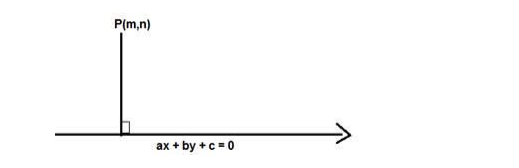# Find the distance of the point (3, -5) from the lineQuestion:

Find the distance of the point $(3,-5)$ from the line $3 x-4 y=27$

Solution:

Given: Point (3,-5) and line 3x – 4y = 27

To find: The distance of the point $(3,-5)$ from the line $3 x-4 y=27$

Formula used:

We know that the distance between a point P(m,n) and a line ax + by + c = 0 is given by,

$D=\frac{|a m+b n+c|}{\sqrt{a^{2}+b^{2}}}$The equation of the line is $3 x-4 y-27=0$

Here $m=3$ and $n=-5, a=3, b=-4, c=-27$

$D=\frac{|3(3)-4(-5)-27|}{\sqrt{3^{2}+4^{2}}}$

$D=\frac{|9+20-27|}{\sqrt{9+16}}=\frac{|29-27|}{\sqrt{25}}=\frac{|2|}{5}$

$D=\frac{2}{5}$

The distance of the point $(3,-5)$ from the line $3 x-4 y=27$ is $\frac{2}{5}$ units